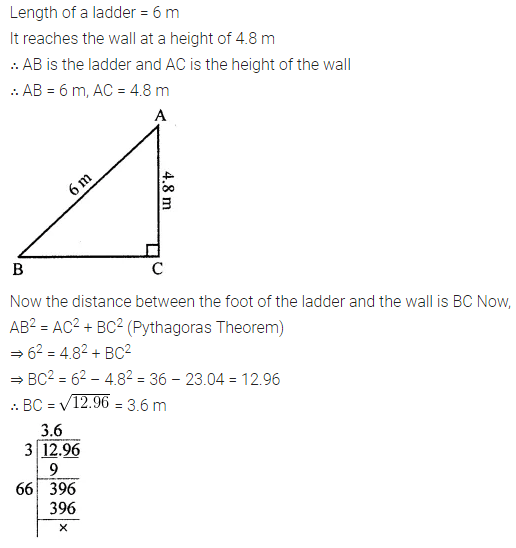# ML Aggarwal Class 8 Solutions for ICSE Maths Chapter 3 Squares and Square Roots Ex 3.4

## ML Aggarwal Class 8 Solutions for ICSE Maths Chapter 3 Squares and Square Roots Ex 3.4

Question 1.
Find the square root of each of the following by division method:
(i) 2401
(ii) 4489
(iii) 106929
(iv) 167281
(v) 53824
(vi) 213444
Solution: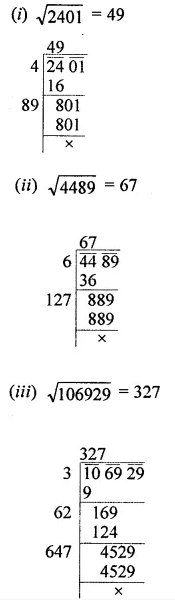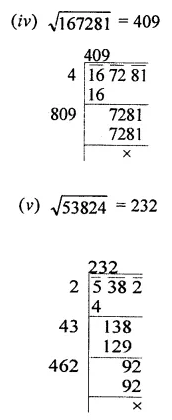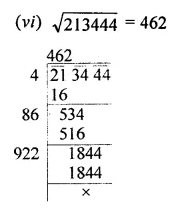Question 2.
Find the number of digits in the square root of each of the following (without any calculation):
(i) 81
(ii) 169
(iii) 4761
(iv) 27889
(v) 525625
Solution:Question 3.
Find the square root of the following decimal numbers by division method:
(i) 51.84
(ii) 42.25
(iii) 18.4041
(iv) 5.774409
Solution: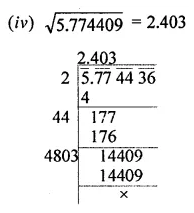Question 4.
Find the square root of the following numbers correct to two decimal places:
(i) 645.8
(ii) 107.45
(iii) 5.462
(iv) 2
(v) 3
Solution: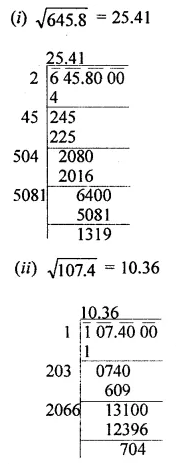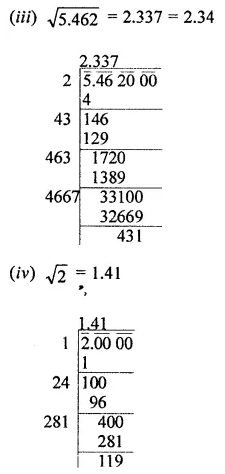Question 5.
Find the square root of the following fractions by division method: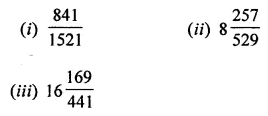Solution: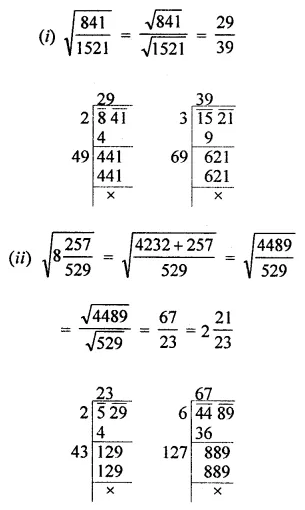Question 6.
Find the least number which must be subtracted from each of the following numbers to make them a perfect square. Also find the square root of the perfect square number so obtained:
(i) 2000
(ii) 984
(iii) 8934
(iv) 11021
Solution: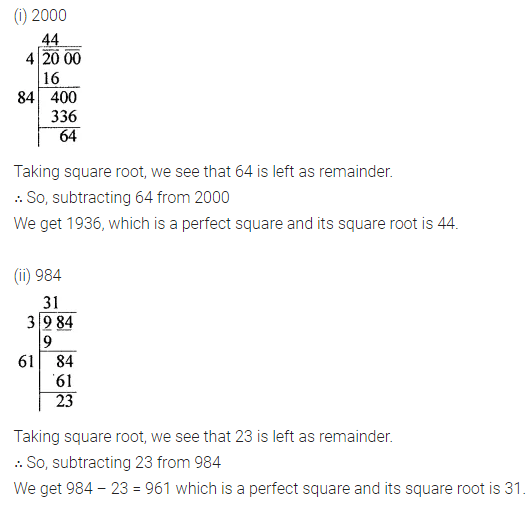Question 7.
Find the least number which must be added to each of the following numbers to make them a perfect square. Also find the square root of the perfect square number so obtained:
(i) 1750
(ii) 6412
(iii) 6598
(iv) 8000
Solution: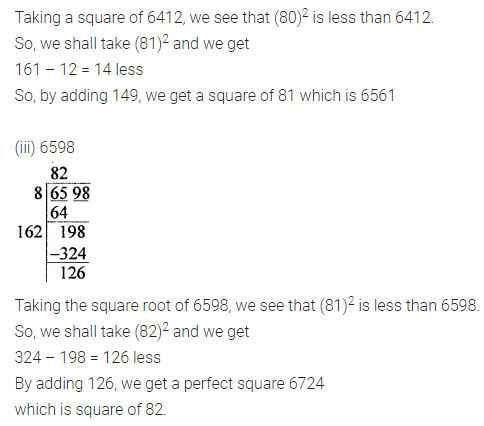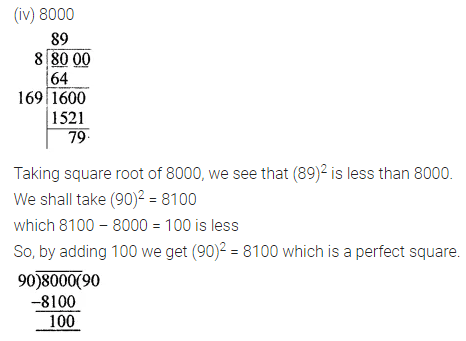Question 8.
Find the smallest four-digit number which is a perfect square.
Solution:Question 9.
Find the greatest number of six digits which is a perfect square.
Solution: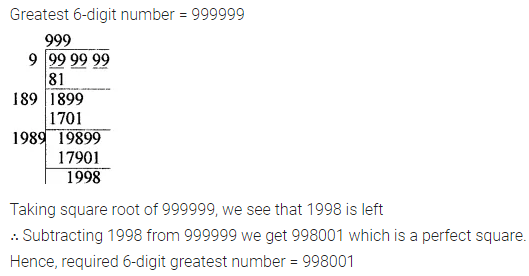Question 10.
In a right triangle ABC, ∠B = 90°.
(i) If AB = 14 cm, BC = 48 cm, find AC.
(ii) If AC = 37 cm, BC = 35 cm, find AB.
Solution: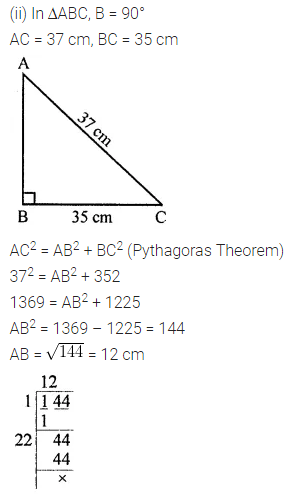Question 11.
A gardener has 1400 plants. He wants to plant these in such a way that the number of rows and number of columns remains the same. Find the minimum number of plants he needs more for this.
Solution:Question 12.
There are 1000 children in a school. For a P.T. drill they have to stand in such a way that the number of rows is equal to a number of columns. How many children would be left out in this arrangement?
Solution: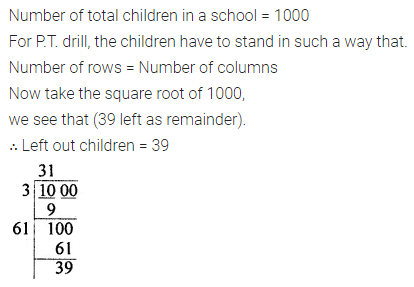Question 13.
Amit walks 16 m south from his house and turns east to walk 63 ra to reach his friend’s house. While returning, he walks diagonally from his friend’s house to reach back to his house. What distance did he walk while returning?
Solution:Question 14.
A ladder 6 m long leaned against a wall. The ladder reaches the wall to a height of 4.8 m. Find the distance between the wall and the foot of the ladder.
Solution: